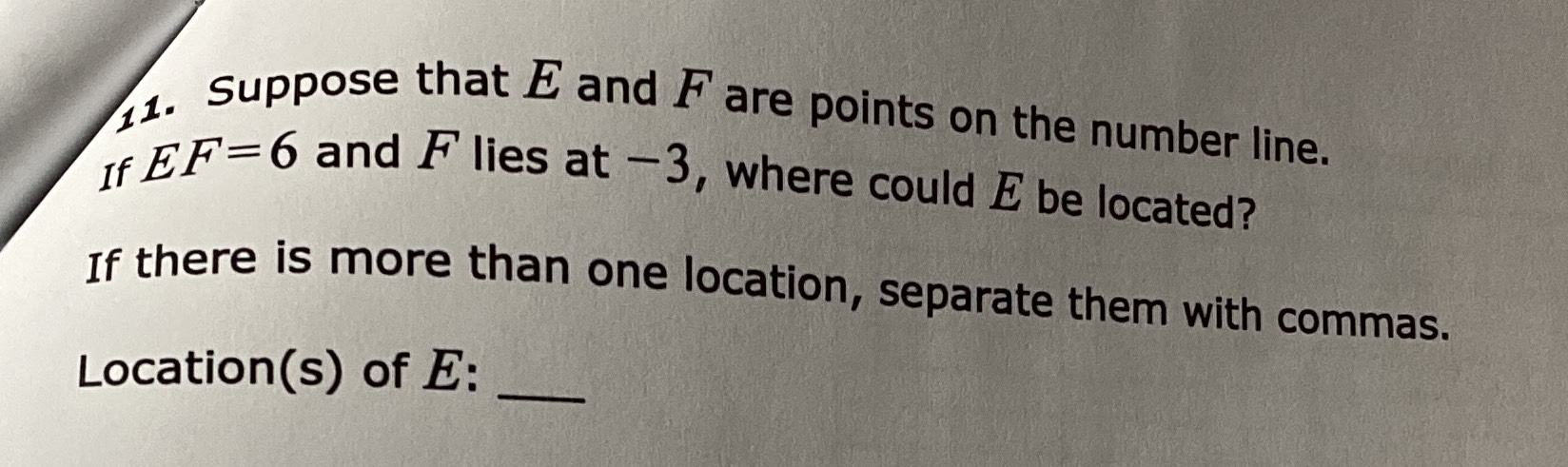### ¿Todavía tienes preguntas de matemáticas?

Pregunte a nuestros tutores expertos
Algebra
Pregunta1. Suppose that $$E$$ and $$F$$ are points on the number line. If $$E F = 6$$ and $$F$$ lies at $$- 3$$ , where could $$E$$ be located? If there is more than one location, separate them with commas. Location(s) of $$E :$$

$$E = 3,\ - 9$$